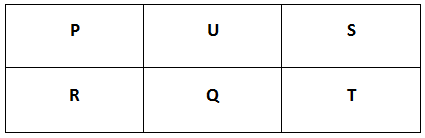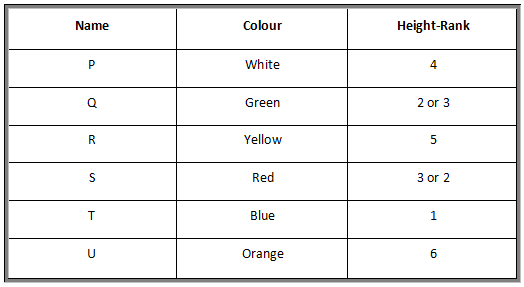### CAT 2008 Question Paper Question 26

Instructions

Directions for the following three questions: Answer the following questions based on the statements given below:

(i) There are three houses on each side of the road.

(ii) These six houses are labeled as P, Q, R, S, T and U.

(iii) The houses are of different colours, namely, Red, Blue, Green, Orange, Yellow and White.

(iv) The houses are of different heights.

(v) T, the tallest house, is exactly opposite to the Red coloured house.

(vi) The shortest house is exactly opposite to the Green coloured house.

(vii) U, the Orange coloured house, is located between P and S.

(viii) R, the Yellow coloured house, is exactly opposite to P.

(ix) Q, the Green coloured house, is exactly opposite to U.

(x) P, the White coloured house, is taller than R, but shorter than S and Q.

Question 26

# What is the colour of the house diagonally opposite to the Yellow coloured house?

Solution

Before directly trying to answer the question, it is important to gather all the information given by the question.

There are three houses on each side of the road => Draw 6 lines, 3 in each row, to accommodate P, Q, R, S, T and U.

The houses are of different colours and different heights.

T is tallest and is opposite to red house => Let’s number T as 1.

Shortest house is opposite to green house.

U is orange and is between P and S => Two cases arise here. P-U-S is one possibility and the other possibility is S-U-P.R is yellow and is opposite to P.

Q is green and is opposite to U. We know that green house is opposite to the shortest house. This implies that U is the shortest house => Number of U is 6.

P is white and is taller than R but shorter than S and Q => Apart from T, S and Q are also taller than P => S and Q can be 2 and 3 in any order => Number of P is 4 and number of R is 5.

We know that P is opposite to R and Q is opposite to U => S is opposite to T

It is given that T is opposite to red house => S is the red house and hence T is the blue house.

So, we know the colours of all houses and heights of P, R, T and U.

In this question, we are asked to find the house that is opposite to yellow house. R is the yellow house, P is opposite to R and S is on the other corner in P’s row. Hence S is the house that is diagonally opposite to yellow house and the colour of S is Red.